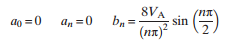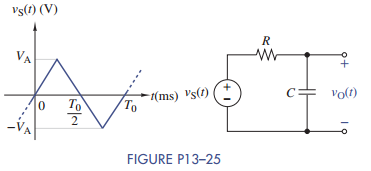### Create an Account

Home / Questions / The periodic triangular wave in Figure P13–25 is applied to the RC circuit shown in the f...

# The periodic triangular wave in Figure P13–25 is applied to the RC circuit shown in the figure. The Fourier coefficients of the input are If VA 10 V and T0 2π ms find the first four

The periodic triangular wave in Figure P13–25 is applied to the RC circuit shown in the figure. The Fourier coefficients of the input areIf VA = 10 V and T0 = 2π ms, find the first four nonzero terms in the Fourier series of vO (t) for R =5kΩ and C = 0.01 μF.May 24 2020 View more View LessSubscribe To Get Solution